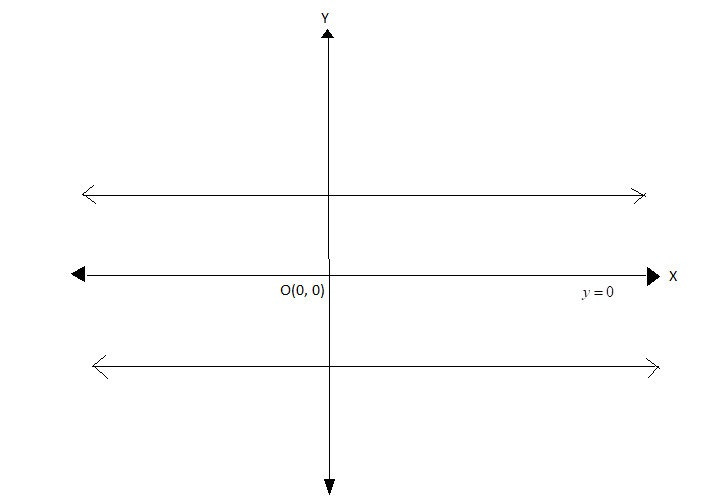Find the equation of a straight line parallel to the $x$- axis.(A) $x = a$(B) $y = a$(C) $y = x$(D) $y = - a$Verified
149.4k+ views
Hint: Express the equation of$x$- axis in the form$Ax + By + C = 0$ and find its slope using the formula $m = \dfrac{{ - A}}{B}$ where m represents the slope.
Use the fact that parallel lines have the same slope to calculate the slope of the line parallel to the $x$- axis. Use this slope and the general equation of the parallel line $Ax + By + {C_1} = 0$ where $C \ne {C_1}$ to get the answer.

We are asked to find the equation of a straight line which is parallel to the $x$- axis.
We know that the equation of the $x$- axis is$y = 0$
This means that any point on the $x$- axis will have its $y -$ coordinate as 0.
Any line which is parallel to the $x$- axis will look like the below figure:That is, the line will lie either above the $x$- axis or below it. Also, it will pass through the $y$- axis.
The general equation of a straight line is given by $Ax + By + C = 0$
Here, the letters A, B, and C are real numbers. Also, A and B are non-zero constants.
Now, the slope of the straight line given by the equation $Ax + By + C = 0$is $m = \dfrac{{ - A}}{B}$ where $B \ne 0$
We will compare the equation of $x$- axis with the general form $Ax + By + C = 0$to obtain its slope.
Now, the equation of $x$- axis is $y = 0$.
Therefore, we can express this equation as $0x + y + 0 = 0$.
Thus on comparison with the general form $Ax + By + C = 0$, we get $A = 0$,$B = 1$ and $C = 0$
Therefore, the slope of the equation of $x$- axis is $m = - \dfrac{0}{1} = 0$
But the question is not about finding the slope or equation of the $x$- axis.
We need the equation of the line parallel to the $x$- axis.
Now, we know that the general equation of any line which is parallel to the line represented by the equation $Ax + By + C = 0$ would be of the form $Ax + By + {C_1} = 0$ where $C \ne {C_1}$ and ${C_1}$ is also a real number.
We can notice here that the coefficients A and B of the variables $x$ and $y$ are the same for a pair of parallel lines.
Therefore, the slope of a line parallel to the line represented by the equation $Ax + By + C = 0$will also be given by $m = \dfrac{{ - A}}{B}$ where $B \ne 0$
Thus, we can conclude that parallel lines have the same slope.
Therefore, the slope of the line parallel to the $x$- axis will be 0 as well.
This is only possible if $A = 0$as$m = \dfrac{{ - A}}{B} = 0 \Rightarrow - A = 0 \Rightarrow A = 0$
Substitute$A = 0$ in the general equation of the parallel line $Ax + By + {C_1} = 0$
This gives us
$0x + By + {C_1} = 0 \\ \Rightarrow By = - {C_1} \\ \Rightarrow y = \dfrac{B}{{ - {C_1}}} = - \dfrac{B}{{{C_1}}} \\$
Let$- \dfrac{B}{{{C_1}}} = a$.
Thus the equation becomes $y = a$
Hence the equation of any line parallel to $x$- axis is $y = a$

Note:
A common mistake made by many students is that they tend to take the coefficient of $x$ in the numerator and that of $y$ in the denominator to calculate the slope. One needs to be careful with this substitution which is the key to answering such questions.